# Drift Equations

The drift equations take the form: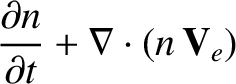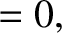(4.200)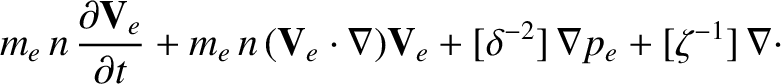(4.201)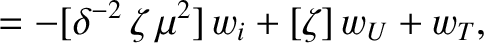(4.202)

and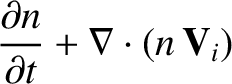(4.203)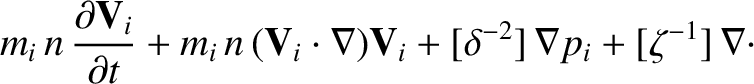(4.204)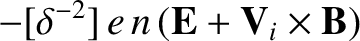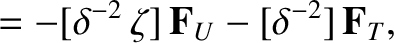(4.205)

If we assume thatthen the dominant term in the electron energy conservation equation (4.202) yields(4.206)

which implies that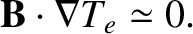(4.207)

In other words, in the drift approximation, the parallel electron heat conductivity is usually sufficiently large that it forces the electron temperature to be constant on magnetic field-lines.

The dominant term in the ion energy conservation equation (4.205) yields(4.208)

which implies that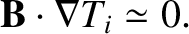(4.209)

In other words, in the drift approximation, the parallel ion heat conductivity is usually sufficiently large that it forces the ion temperature to be constant on magnetic field-lines.

The dominant terms in the electron and ion momentum conservation equations, (4.201) and (4.204), yield(4.210)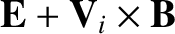(4.211)

The sum of the preceding two equations gives(4.212)

where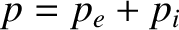. In other words, in the drift approximation, to lowest order, the plasma exists in an equilibrium state in which the magnetic force density balances the total scalar pressure force density. It follows from the previous equation that(4.213)

In other words, in the drift approximation, the total plasma pressure is constant along magnetic field-lines. Given that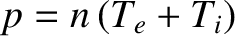, and making use of Equations (4.207) and (4.209), we deduce that(4.214)

In other words, in the drift approximation, the electron number density is constant along magnetic field-lines. It follows that the electron and ion pressures,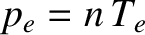and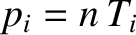, are also constant along magnetic field-lines. Hence, Equation (4.210) yields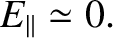(4.215)

In other words, in the drift approximation, to lowest order, the parallel electric field is zero.

Equations (4.210)–(4.211) can be inverted to give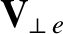(4.216)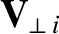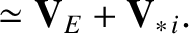(4.217)

Here,is the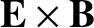velocity, whereas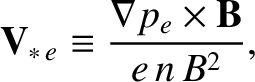(4.218)

and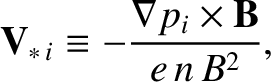(4.219)

are termed the electron diamagnetic velocity and the ion diamagnetic velocity, respectively.

According to Equations (4.216)–(4.217), in the drift approximation, the velocity of the electron fluid perpendicular to the magnetic field is the sum of thevelocity and the electron diamagnetic velocity. A similar statement can be made for the ion fluid. By contrast, in the MHD approximation the perpendicular velocities of the two fluids consist of thevelocity alone, and are, therefore, identical to lowest order. The main difference between the two orderings lies in the assumed magnitude of the electric field. In the MHD limit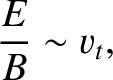(4.220)

whereas in the drift limit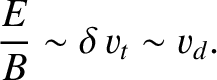(4.221)

Thus, the MHD ordering can be regarded as a strong electric field ordering, whereas the drift ordering corresponds to a weak electric field ordering.

The diamagnetic velocities are so named because the diamagnetic current,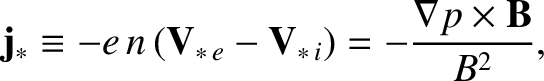(4.222)

generally acts to reduce the magnitude of the magnetic field inside the plasma.

The electron diamagnetic velocity can be written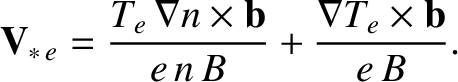(4.223)

In order to account for this velocity, let us consider a simplified case in which the electron temperature is uniform, there is a uniform density gradient running along the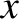-direction, and the magnetic field is parallel to the-axis. (See Figure 4.3.) The electrons gyrate in the-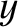plane in circles of radius. At a given point, coordinate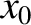, say, on the-axis, the electrons that come from the right and the left have traversed distances of approximate magnitude. Thus, the electrons from the right originate from regions where the particle density is approximately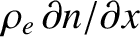greater than the regions from which the electrons from the left originate. It follows that the-directed particle flux is unbalanced, with slightly more particles moving in the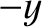-direction than in the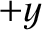-direction. Thus, there is a net particle flux in the-direction: that is, in the direction of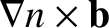. The magnitude of this flux is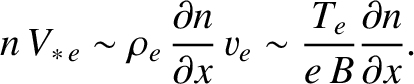(4.224)

There is no unbalanced particle flux in the-direction, because the-directed fluxes are associated with electrons that originate from regions where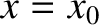. We have now accounted for the first term on the right-hand side of Equation (4.223). We can account for the second term using similar arguments. The ion diamagnetic velocity is similar in magnitude to the electron diamagnetic velocity, but is oppositely directed, because ions gyrate in the opposite direction to electrons.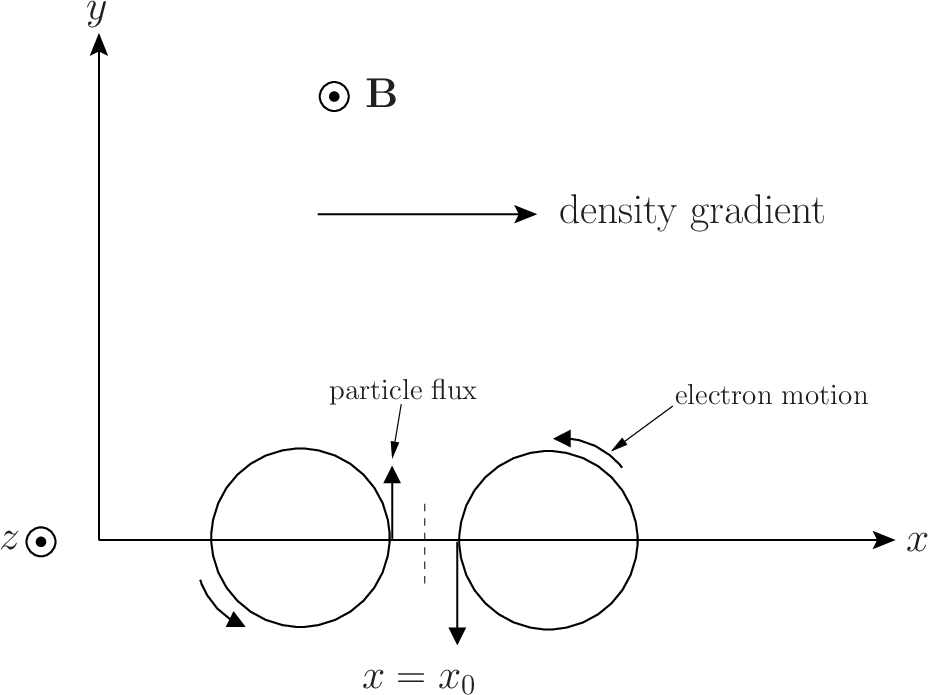The most curious aspect of diamagnetic flows is that they represent fluid flows for which there is no corresponding motion of the particle guiding centers. Nevertheless, the diamagnetic velocities are real fluid velocities, and the associated diamagnetic current is a real current. For instance, the diamagnetic current contributes to force balance inside the plasma, and also gives rise to ohmic heating.